New to Qlik Sense

If you’re new to Qlik Sense, start with this Discussion Board and get up-to-speed quickly.

Announcements
QlikWorld 2022, LIVE in Denver CO., May 16-19, 2022. REGISTER NOW TO RECEIVE EARLY BIRD PRICING
cancel
Showing results for
Did you mean: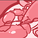Contributor III

Ignore Dimension

Hello to everyone.

I need to create a LINE CHART in the following way:

X dimension: MR_DATE (i.e. month+year)

2° dimension (different lines):  CATEGORY (I need to split my measure by the different categories)

Measure: Count( ITEM ) / sum( IB )

My problem is the following: while for the Count( ITEM ) is necessary to have different values according the different CATEGORY values, I need that sum ( IB ) is independent from the CATEGORY dimension (i.e. for all the CATEGORY values the sum( IB ) lines has to be all equal to each other).

What I need (all sum (IB) lines overlapped - no changes from different CATEGORY value):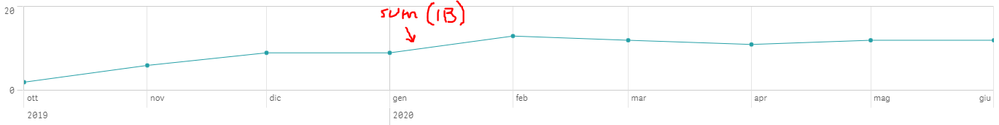What I currently get: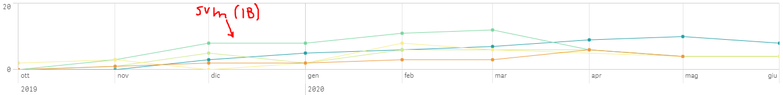I'm supposing this depends from the fact that for some CATEGORY values, some component of the IB are not existing (for example the sum (IB) is summing the IB from different countries and if for a specific CATEGORY and MR_DATE a country is missing, I'm losing it's contribution to the total IB).

Currently, since the sum ( IB ) should be influenced only by the following fields: MR_DATE and COUNTRY, I'm using the following formula:

sum( {1<COUNTRY = \$::COUNTRYMR_DATE = \$::MR_DATE >} [IB] )

I was trying to ignore all the selections but COUNTRY and MR_DATE with the aim to ignore also CATEGORY... however it doesn't work => the lines in the graph are different according to the specific CATEGORY...

How can I ignore, only for the sum( IB ) the split between the different CATEGORIES, keeping it for the count( ITEM) ?

Thanks!

Eugenio

Labels (3)

• selections

4 RepliesPartner

hi

you should use this expression :
sum( {1<COUNTRY = \$::COUNTRY ,  MR_DATE = \$::MR_DATE >}  total <MR_DATE> [IB] )Contributor III
Author

I've tried to implement the solution and the result was close to what I was searching, but:

Result expected: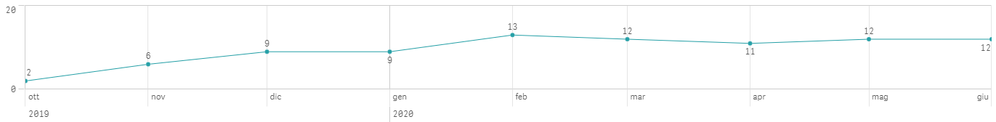Result obtained using the proposed solution: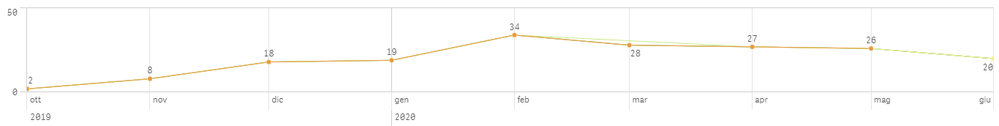1) The values of the points are different from the expected (Oct 2019 is ok < 2 in both >, but from Nov 2019 the values are quite different...

2) For some dates (i.e. Mar 2020) the points for some CATEGORY values are missing (straight line from adjacent months)

Any idea on how to manage these 2 situations?

Thanks!

EugenioPartner

hi

in order to solve this issue ,

can you share some sample dataContributor III
Author

Thanks!

I've uploaded the .qvf file with the 2 graphs.

Regards

EugenioTags
Community Browser